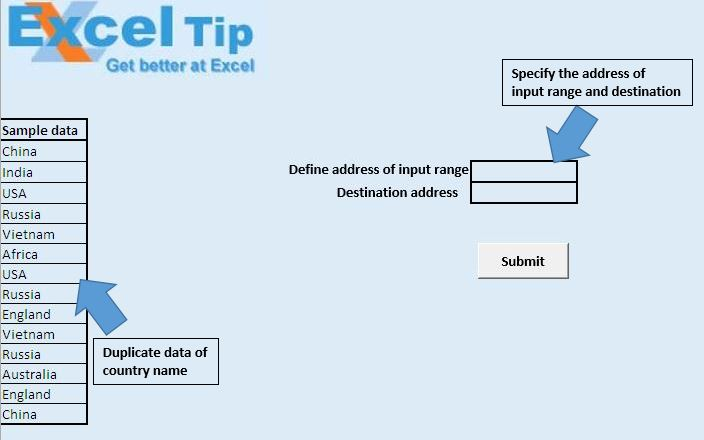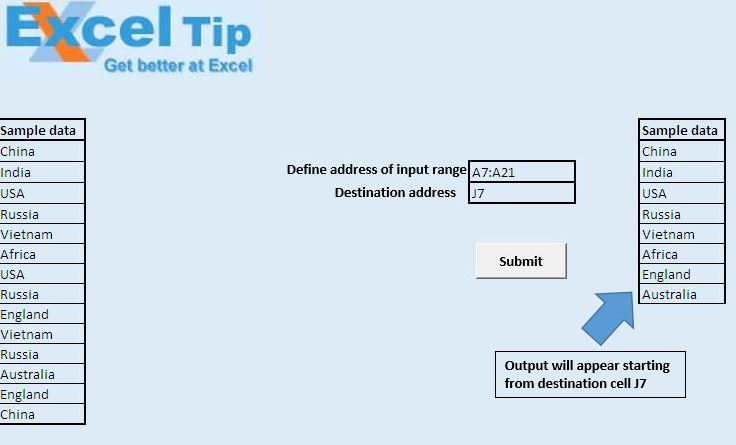# Return unique items using VBA in Microsoft Excel

In this article, we will create a macro to extract unique values from the defined range.

Raw data for this example consists of duplicate entries of country names in the range A7:A21.We have created “FindUniqueValues” macro to extract unique values from the defined range. This macro can be run by clicking the “Submit” button. Before clicking the “Submit” button, we have to specify the address of the range which contains duplicate data in the cell H9 and address of the destination where output should appear.Logic explanation

“FindUniqueValues” macro takes two range objects as input parameters. First parameter defines the range which contains the duplicate data and second parameter defines the position of the starting cell which will contain the output. This macro cannot be called directly as we have to specify the parameters, so we have created second macro “MacroRunning” to call the macro.

“MacroRunning” macro calls the “FindUniqueValues” macro with the parameter input by the user.

Code explanation

CopyToRange:=TargetCell, Unique:=True

AdvancedFilter method of Range object is used to filter or copy data from the range based on a certain criteria. To copy only the unique values, we have to set Unique parameter of AdvancedFilter to True.

```
Option Explicit

Sub FindUniqueValues(SourceRange As Range, TargetCell As Range)

'Using advance filter for extacting unique items in the source range
CopyToRange:=TargetCell, Unique:=True

End Sub

Sub MacroRunning()

'Calling FindUniqueValues macro
Call FindUniqueValues(Range(Range("H9").Value), Range(Range("H10").Value))

End Sub

```

We would love to hear from you, do let us know how we can improve our work and make it better for you. Write to us at info@exceltip.com

1."This function was missing from my previous post:

Function ArrayDimensionCount(InputArray As Variant) As Long
' returns the number of dimensions of InputArray
Dim i As Long, d As Long
If Not TypeName(InputArray) Like ""*()"" Then Exit Function ' return 0
On Error Resume Next
i = 0
Do
i = i + 1
d = UBound(InputArray, i)
Loop Until Err 0
Err = 0
On Error GoTo 0
ArrayDimensionCount = i - 1 ' return the dimension count
End Function "

2."There are many possibilities here. One solutions is the following:

Sub ExampleMultipleRanges()
' returnes the unique items from A1:A10;C1:C10 to E1 and downwards
Dim varArray As Variant
varArray = ReturnUniqueItems(True, Range(""A1:A10""), Range(""C1:C10""))
ArrayToRange varArray, Range(""E1"")
Erase varArray
End Sub

Function ReturnUniqueItems(blnReturnItems As Boolean, ParamArray InputRange()) As Variant
' returns the unique items or the count of unique items from a single range or multiple ranges
Dim c As Collection, i As Long, cl As Range, arrResult() As Variant
ReturnUniqueItems = CVErr(xlErrNA)
Set c = New Collection
For i = LBound(InputRange) To UBound(InputRange)
For Each cl In InputRange(i)
If Len(cl.Text) > 0 Then
On Error Resume Next
c.Add cl.Value, cl.Text ' ignores duplicates
On Error GoTo 0
End If
Next cl
Set cl = Nothing
Next i
If blnReturnItems Then ' return each unique item
If c.Count > 0 Then
ReDim arrResult(0 To c.Count - 1)
For i = 1 To c.Count
arrResult(i - 1) = c(i)
Next i
End If
'ReturnUniqueItems = arrResult ' return data as a single row
ReturnUniqueItems = Application.Transpose(arrResult) ' return data as a single column
' the transpose function can handle up to 5461 items
Erase arrResult
Else ' return the count of unique items
ReturnUniqueItems = c.Count
End If
Set c = Nothing
End Function

Sub ArrayToRange(InputArray As Variant, UpperLeftCell As Range)
' fills the content of InputArray into a cell range starting at UpperLeftCell
Dim d As Long, r As Long, c As Long
If UpperLeftCell Is Nothing Then Exit Sub
If Not TypeName(InputArray) Like ""*()"" Then Exit Sub
d = ArrayDimensionCount(InputArray)
If d 2 Then Exit Sub
Select Case d
Case 1 ' 1-dimensional array
r = UBound(InputArray, 1)
c = 1
Case 2 ' 2-dimensional array
r = UBound(InputArray, 1)
c = UBound(InputArray, 2)
End Select
UpperLeftCell.Resize(r, c).Formula = InputArray
End Sub "

3.What about taking this further and finding unique items from multiple ranges

4."SourceRange and TargetCell can be on different worksheets, e.g. like this:
FindUniqueValues Worksheets(1).Range(""A2:A100""), Worksheets(2).Range(""C1"") "

5.A good way of getting a unique list, but note that SourceRange and TargetCell must be on the same worksheet.

6."This function was missing from my previous post:

Function ArrayDimensionCount(InputArray As Variant) As Long
' returns the number of dimensions of InputArray
Dim i As Long, d As Long
If Not TypeName(InputArray) Like ""*()"" Then Exit Function ' return 0
On Error Resume Next
i = 0
Do
i = i + 1
d = UBound(InputArray, i)
Loop Until Err 0
Err = 0
On Error GoTo 0
ArrayDimensionCount = i - 1 ' return the dimension count
End Function "

7."There are many possibilities here. One solutions is the following:

Sub ExampleMultipleRanges()
' returnes the unique items from A1:A10;C1:C10 to E1 and downwards
Dim varArray As Variant
varArray = ReturnUniqueItems(True, Range(""A1:A10""), Range(""C1:C10""))
ArrayToRange varArray, Range(""E1"")
Erase varArray
End Sub

Function ReturnUniqueItems(blnReturnItems As Boolean, ParamArray InputRange()) As Variant
' returns the unique items or the count of unique items from a single range or multiple ranges
Dim c As Collection, i As Long, cl As Range, arrResult() As Variant
ReturnUniqueItems = CVErr(xlErrNA)
Set c = New Collection
For i = LBound(InputRange) To UBound(InputRange)
For Each cl In InputRange(i)
If Len(cl.Text) > 0 Then
On Error Resume Next
c.Add cl.Value, cl.Text ' ignores duplicates
On Error GoTo 0
End If
Next cl
Set cl = Nothing
Next i
If blnReturnItems Then ' return each unique item
If c.Count > 0 Then
ReDim arrResult(0 To c.Count - 1)
For i = 1 To c.Count
arrResult(i - 1) = c(i)
Next i
End If
'ReturnUniqueItems = arrResult ' return data as a single row
ReturnUniqueItems = Application.Transpose(arrResult) ' return data as a single column
' the transpose function can handle up to 5461 items
Erase arrResult
Else ' return the count of unique items
ReturnUniqueItems = c.Count
End If
Set c = Nothing
End Function

Sub ArrayToRange(InputArray As Variant, UpperLeftCell As Range)
' fills the content of InputArray into a cell range starting at UpperLeftCell
Dim d As Long, r As Long, c As Long
If UpperLeftCell Is Nothing Then Exit Sub
If Not TypeName(InputArray) Like ""*()"" Then Exit Sub
d = ArrayDimensionCount(InputArray)
If d 2 Then Exit Sub
Select Case d
Case 1 ' 1-dimensional array
r = UBound(InputArray, 1)
c = 1
Case 2 ' 2-dimensional array
r = UBound(InputArray, 1)
c = UBound(InputArray, 2)
End Select
UpperLeftCell.Resize(r, c).Formula = InputArray
End Sub "

8.What about taking this further and finding unique items from multiple ranges

9."SourceRange and TargetCell can be on different worksheets, e.g. like this:
FindUniqueValues Worksheets(1).Range(""A2:A100""), Worksheets(2).Range(""C1"") "

10.A good way of getting a unique list, but note that SourceRange and TargetCell must be on the same worksheet.

Terms and Conditions of use

The applications/code on this site are distributed as is and without warranties or liability. In no event shall the owner of the copyrights, or the authors of the applications/code be liable for any loss of profit, any problems or any damage resulting from the use or evaluation of the applications/code.